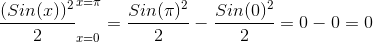Calculus 1 : Area

Example Questions

Example Question #1 : How To Graph Functions Of Area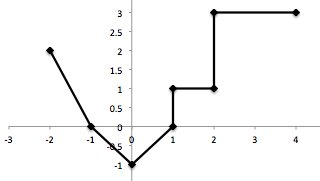Graph of a piecewise-linear function, for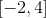, is shown above.

Find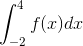.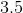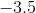Explanation:

Find the area under the graphfrom. To do this break the graph up into triangles, squares, and rectangles to calculate the individual areas over smaller intervals and then add them all together.

The areas are added to be: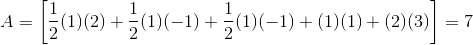Therefore,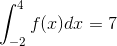Example Question #2 : How To Graph Functions Of Area

Find the area bounded by the curve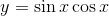and the-axis over the interval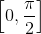.Explanation:

The curve is in quadrant one over the given interval, which gives us the bounds of integration.  Evaluating this definite integral yields the area we are after.

In order to performe the antiderivative, let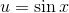.  It follows that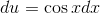.  Therefore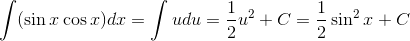so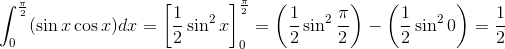Example Question #3 : How To Graph Functions Of Area

Find the area bounded by the curve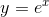and the-axis over the interval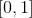.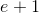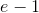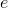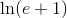Explanation:

The curve is positive over the given interval, so the endpoints of the interval will mark the bounds of integration.  This function is very easy to integrate because the derivative of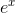is itself!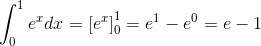Example Question #4 : How To Graph Functions Of Area

Find the area bounded by the curve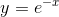and the-axis over the interval.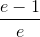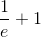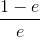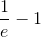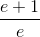Explanation:

This function is positve over the given interval, so the endpoints of the interval mark the bounds of integration.  It is a straightforward integration that is solvable with u-substitution.  Let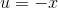so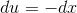.  This means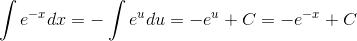so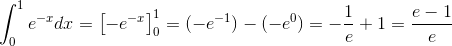Example Question #5 : How To Graph Functions Of Area

Find the area bounded by the curve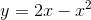in the first quadrant.Explanation:

The curve is in quadrant one over the interval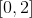, which are the bounds of integration.  To see this, note that the x-intercepts are 0 and 2 and the parabola opens downward.

The definite integral below is solved by taking the antiderivative of each term of the given polynomial function, evaluating this antiderivative at the bounds of integration, and subtracting the values.

For this particular integral use the rule,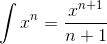to solve.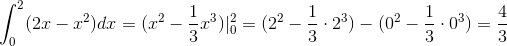Example Question #6 : How To Graph Functions Of Area

Find the area under the curve between the following bounderies of the following function.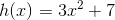in between the boundaries ofand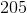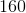Explanation:

We can find the area under the curve by taking the anti-derivative of the function and using the two boundaries as x values.  The anti-derivative ofis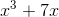.  If we use the two boundaries, we end up with our answer,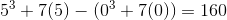.

Example Question #7 : How To Graph Functions Of Area

Evalute the following Definite Integral: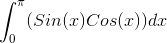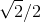Explanation:

For this integral one may be tempted to directly integrate; however, this is no rule of integrals which allows us to do so.

We must apply a complex method of integration, here u-subtitution works best.

We notice that Cos(x) is the derivative of Sin(x) so it may be best to let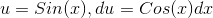.

Now that we have found a proper u and du, we may directly substiute into our original integral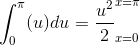However, our limits are in terms of x so we must substitute our u=Sin(x) back in before evaluating